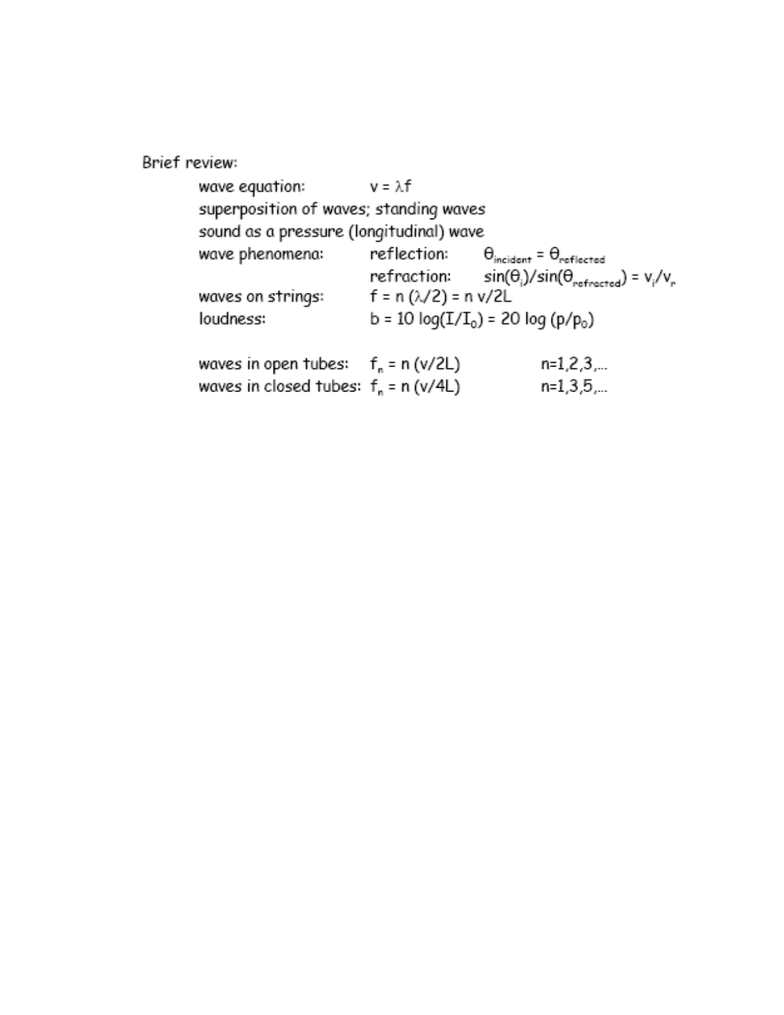Class Notes (1,100,000)
CA (620,000)
McGill (30,000)
Physics (500)
Lecture

# PHYS 101 Lecture Notes - Fundamental Frequency

Department
Physics
Course Code
PHYS 101
Professor
Kenneth Ragan

This preview shows half of the first page. to view the full 3 pages of the document.Phys 101 Alanna Houston
November 13, 2007
SOUND
-
-Example: The voice of a person who has inhaled Helium sounds
very high pitched. Why?
oLook at wave equation. Velocity increases by three and the
frequency increases because the wavelength remains
unchanged
-Example: At 20 degrees C, (take v = 343 m/s), how long an organ
pipe do you need for fundamental to be 294 Hz?
o294 = (2)(343/L)
oL = 0.58 m
-Example: An organ pipe resonates at 264 Hz. 440 Hz, and 616 Hz
but not at any frequencies in between. Is it an open or a closed
pipe?
oClosed pipe. This is because there is a gap of 176, and then
if you subtract 176 from 276, you get 88, which is not the
other. Half of step between harmonics.
oWhat is the fundamental frequency? 88 Hz
Interference:
-Sound waves come together to form interference
-Spatial interference (same region) can happen constructively or
destructively
-Usually only specific wavelengths can interfere destructively
-Interference can also happen in time such as in beats where there
are two waves of similar frequency but not the same frequency.
###### You're Reading a Preview

Unlock to view full version